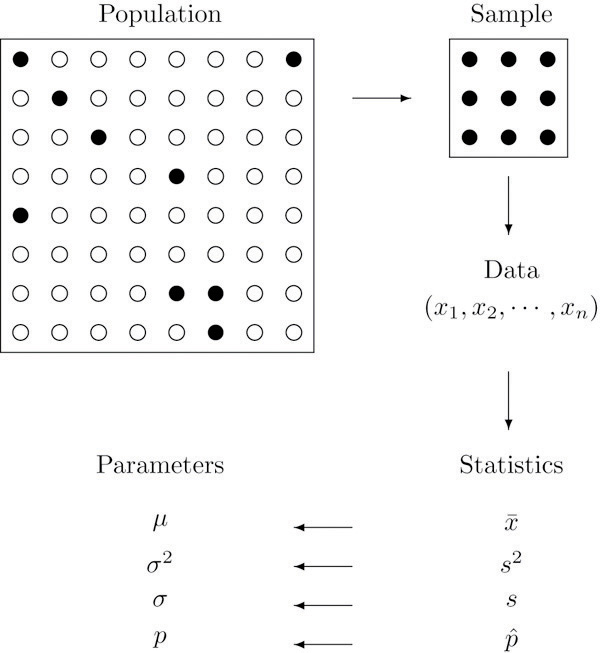There are millions of passenger automobiles in the United States. What is their average value? It is obviously impractical to attempt to solve this problem directly by assessing the value of every single car in the country, adding up all those numbers, and then dividing by however many numbers there are. Instead, the best we can do would be to estimate the average. One natural way to do so would be to randomly select some of the cars, say 200 of them, ascertain the value of each of those cars, and find the average of those 200 numbers. The set of all those millions of vehicles is called the population of interest, and the number attached to each one, its value, is a measurement. The average value is a parameter: a number that describe  a characteristic of the population, in this case monetary worth. The set of 200 cars selected from the population is called a sample, and the 200 numbers, the monetary values of the cars we selected, are the sample data. The average of the data is called a statistic: a number calculated from the sample data. This example illustrates the meaning of the following definitions.

### Definition

A population is any specific collection of objects of interest. A sample is any subset or subcollection of the population, including the case that the sample consists of the whole population, in which case it is termed a census.

### Definition

A measurement is a number or attribute computed for each member of a population or of a sample. The measurements of sample elements are collectively called the sample data.

### Definition

A parameter is a number that summarizes some aspect of the population as a whole. Astatistic is a number computed from the sample data.

Continuing with our example, if the average value of the cars in our sample was $8,357, then it seems reasonable to conclude that the average value of all cars is about$8,357. In reasoning this way we have drawn an inference about the population based on information obtained from the sample. In general,statistics is a study of data: describing properties of the data, which is called descriptive statistics, and drawing conclusions about a population of interest from information extracted from a sample, which is called inferential statistics. Computing the single number \$8,357 to summarize the data was an operation of descriptive statistics; using it to make a statement about the population was an operation of inferential statistics.

### Definition

Statistics is a collection of methods for collecting, displaying, analyzing, and drawing conclusions from data.

### Definition

Descriptive statistics is the branch of statistics that involves organizing, displaying, and describing data.

### Definition

Inferential statistics is the branch of statistics that involves drawing conclusions about a population based on information contained in a sample taken from that population.

The measurement made on each element of a sample need not be numerical. In the case of automobiles, what is noted about each car could be its color, its make, its body type, and so on. Such data are categorical or qualitative, as opposed to numerical or quantitative data such as value or age. This is a general distinction.

### Definition

Qualitative data are measurements for which there is no natural numerical scale, but which consist of attributes, labels, or other nonnumerical characteristics.

### Definition

Quantitative data are numerical measurements that arise from a natural numerical scale.

Qualitative data can generate numerical sample statistics. In the automobile example, for instance, we might be interested in the proportion of all cars that are less than six years old. In our same sample of 200 cars we could note for each car whether it is less than six years old or not, which is a qualitative measurement. If 172 cars in the sample are less than six years old, which is 0.86 or 86%, then we would estimate the parameter of interest, the population proportion, to be about the same as the sample statistic, the sample proportion, that is, about 0.86.

The relationship between a population of interest and a sample drawn from that population is perhaps the most important concept in statistics, since everything else rests on it. This relationship is illustrated graphically in Figure 1.1 “The Grand Picture of Statistics”. The circles in the large box represent elements of the population. In the figure there was room for only a small number of them but in actual situations, like our automobile example, they could very well number in the millions. The solid black circles represent the elements of the population that are selected at random and that together form the sample. For each element of the sample there is a measurement of interest, denoted by a lower case x(which we have indexed as x1,,xnx1,…,xn to tell them apart); these measurements collectively form the sample data set. From the data we may calculate various statistics. To anticipate the notation that will be used later, we might compute the sample mean xx- and the sample proportion pˆp^, and take them as approximations to the population mean μ (this is the lower case Greek letter mu, the traditional symbol for this parameter) and the population proportion p, respectively. The other symbols in the figure stand for other parameters and statistics that we will encounter.

Figure 1.1 The Grand Picture of StatisticsBy the end of this course, you shall know a whole lot about statistics.

### Course Curriculum

No curriculum found !

## N.A

ratings
• 5 stars0
• 4 stars0
• 3 stars0
• 2 stars0
• 1 stars0

No Reviews found for this course.